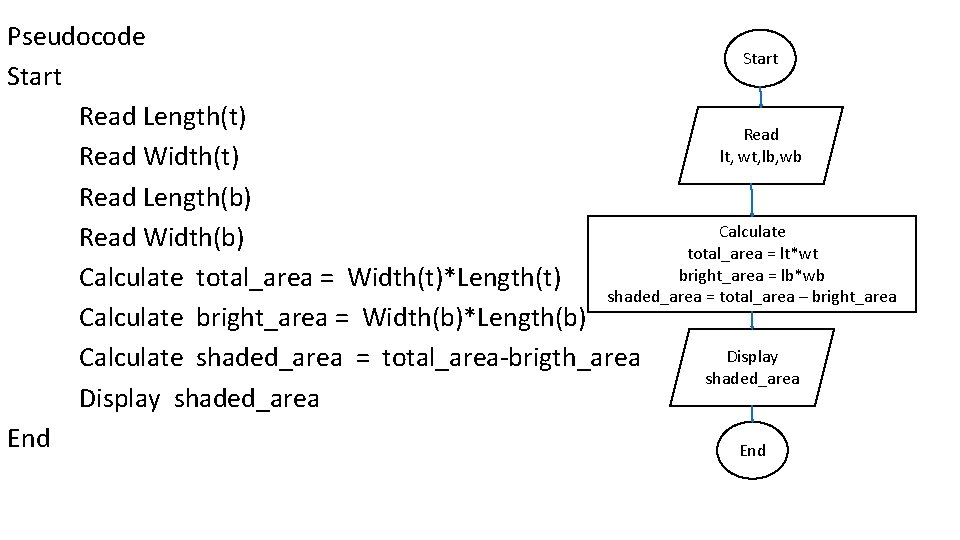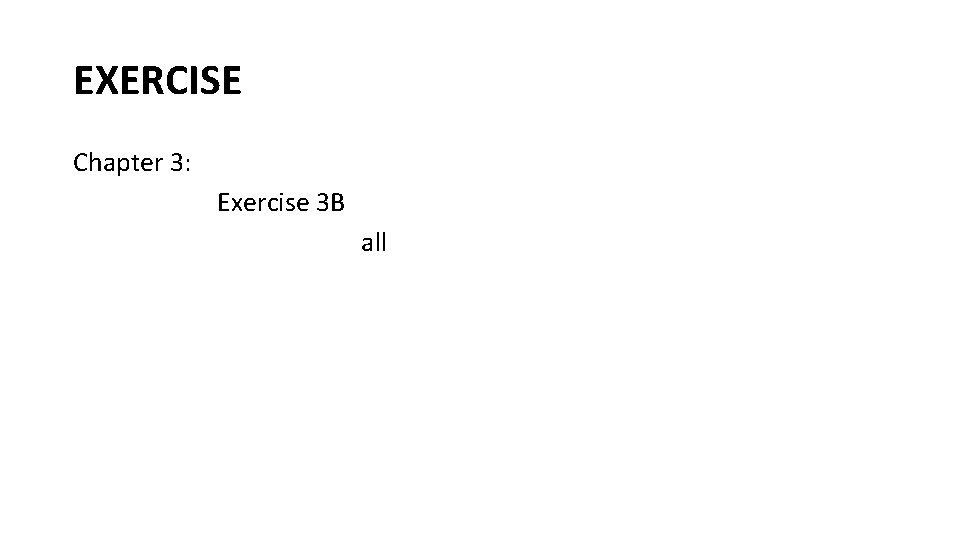# Basic Concepts CONCEPTS of Basic of Algorithm ALGORITHM

• Slides: 34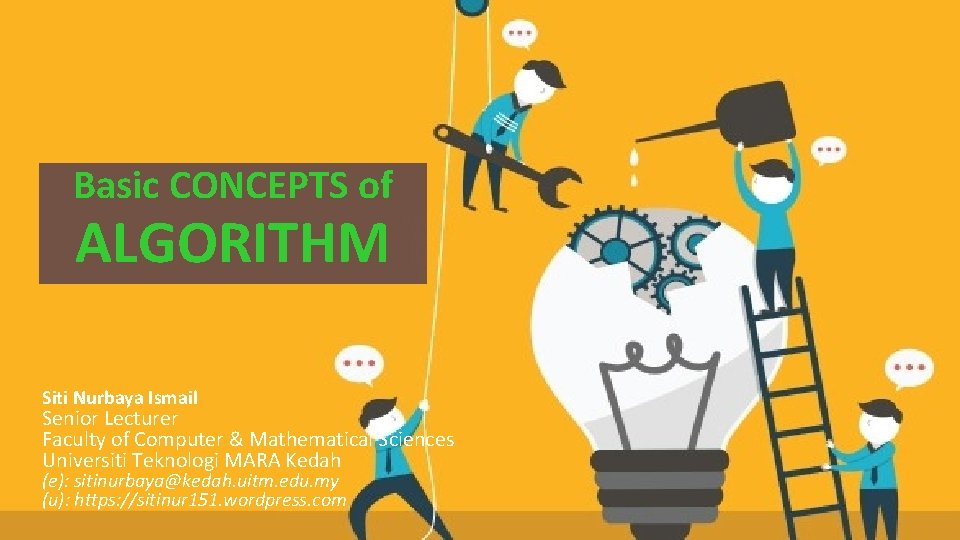Basic. Concepts CONCEPTS of Basic of Algorithm ALGORITHM Siti Nurbaya Ismail Senior Lecturer Faculty of Computer & Mathematical Sciences Universiti Teknologi MARA Kedah (e): [email protected] uitm. edu. my (u): https: //sitinur 151. wordpress. com 1Previously… A program MUST be systematically and properly designed before coding begins. This design process results in the construction of an ALGORITHM.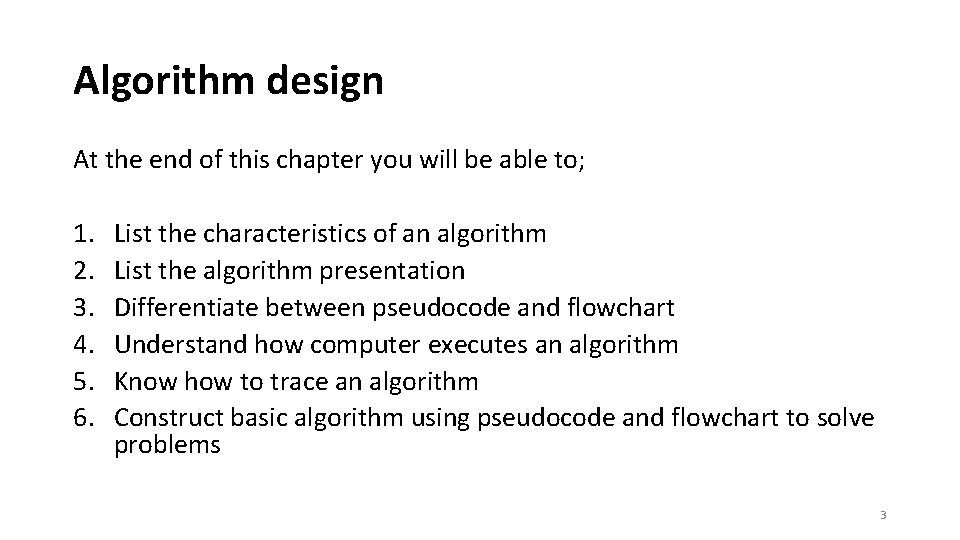Algorithm design At the end of this chapter you will be able to; 1. 2. 3. 4. 5. 6. List the characteristics of an algorithm List the algorithm presentation Differentiate between pseudocode and flowchart Understand how computer executes an algorithm Know how to trace an algorithm Construct basic algorithm using pseudocode and flowchart to solve problems 3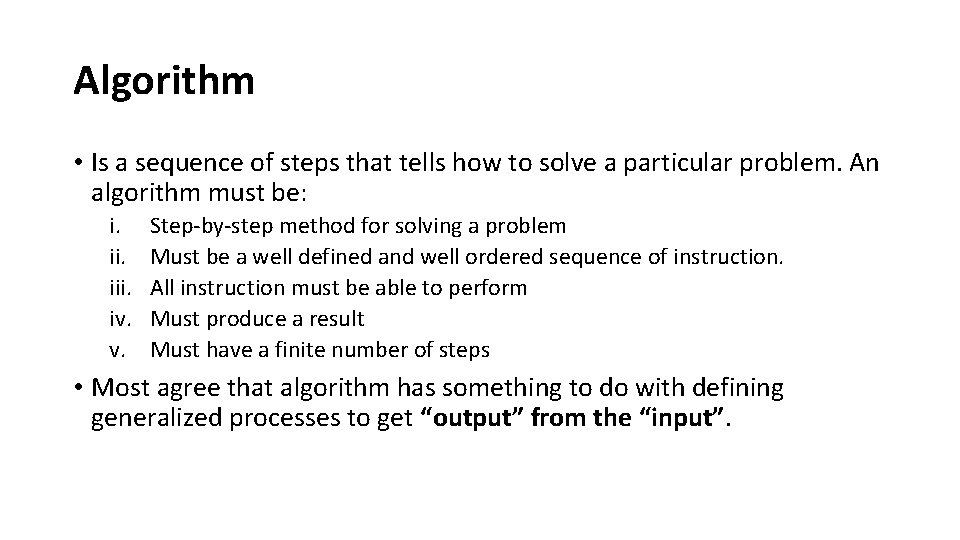Algorithm • Is a sequence of steps that tells how to solve a particular problem. An algorithm must be: i. iii. iv. v. Step‐by‐step method for solving a problem Must be a well defined and well ordered sequence of instruction. All instruction must be able to perform Must produce a result Must have a finite number of steps • Most agree that algorithm has something to do with defining generalized processes to get “output” from the “input”.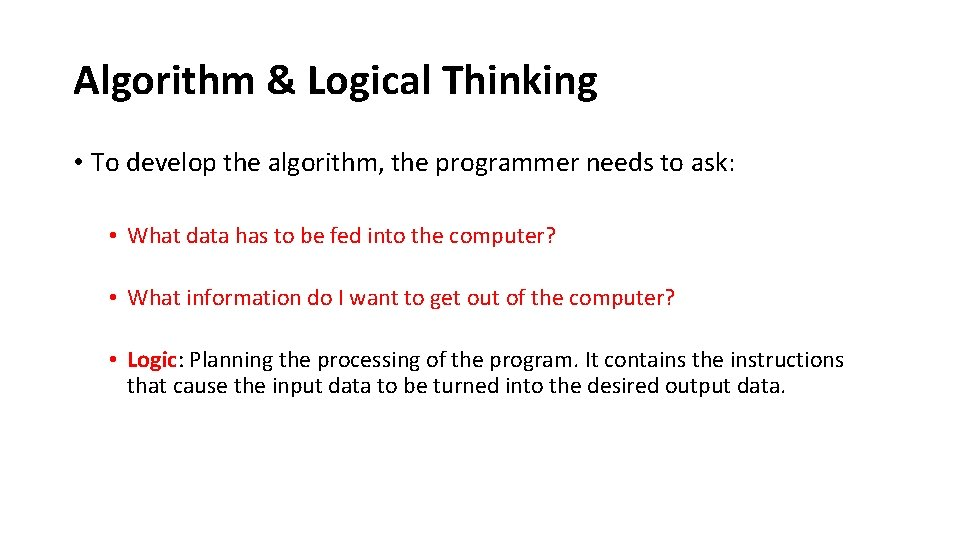Algorithm & Logical Thinking • To develop the algorithm, the programmer needs to ask: • What data has to be fed into the computer? • What information do I want to get out of the computer? • Logic: Planning the processing of the program. It contains the instructions that cause the input data to be turned into the desired output data.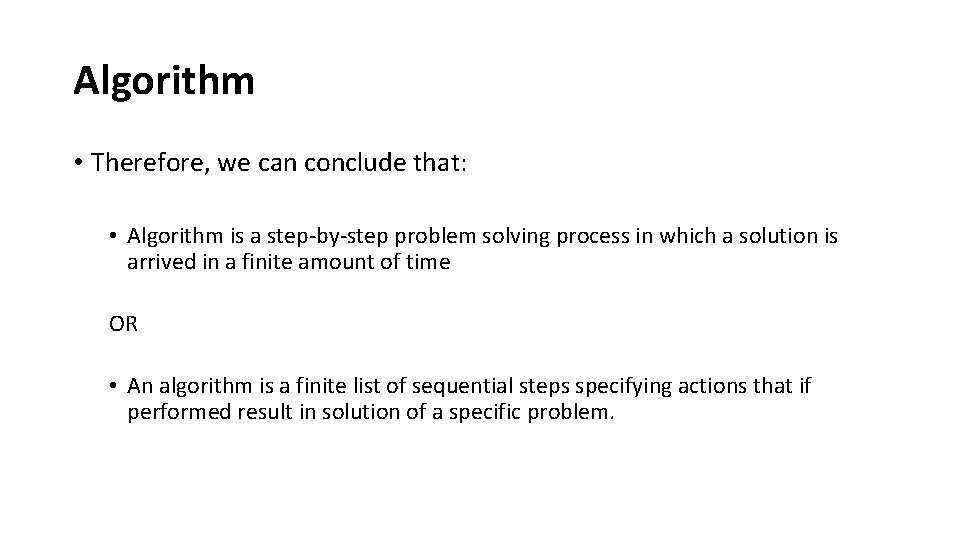Algorithm • Therefore, we can conclude that: • Algorithm is a step‐by‐step problem solving process in which a solution is arrived in a finite amount of time OR • An algorithm is a finite list of sequential steps specifying actions that if performed result in solution of a specific problem.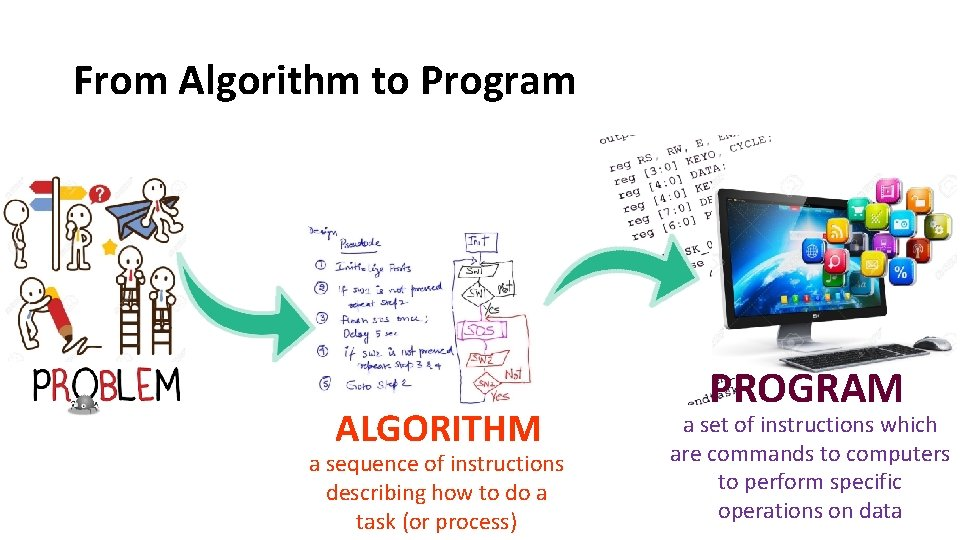From Algorithm to Program ALGORITHM a sequence of instructions describing how to do a task (or process) PROGRAM a set of instructions which are commands to computers to perform specific operations on data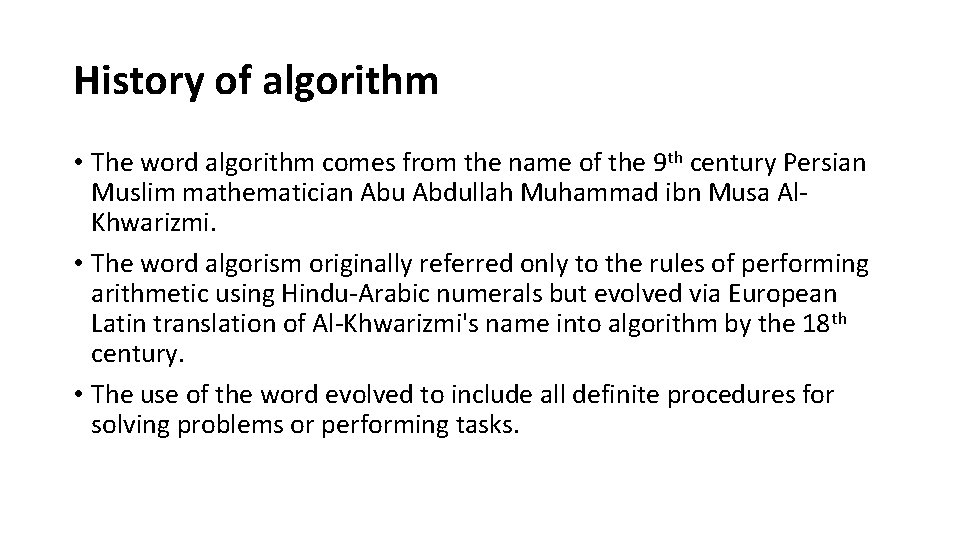History of algorithm • The word algorithm comes from the name of the 9 th century Persian Muslim mathematician Abu Abdullah Muhammad ibn Musa Al‐ Khwarizmi. • The word algorism originally referred only to the rules of performing arithmetic using Hindu‐Arabic numerals but evolved via European Latin translation of Al‐Khwarizmi's name into algorithm by the 18 th century. • The use of the word evolved to include all definite procedures for solving problems or performing tasks.Algorithm Representation • Two types of representation: • Graphically – flowchart • Textual – pseudocode i. Flowchart • Graphical or symbolic representation to present steps in algorithm. • Each symbol has a specific meaning containing a short description of a step. • The flowchart symbols are linked together with arrows showing flow direction ii. Pseudo code • • consist of short English phrases used to describe steps in algorithm. Start with start or begin and ends with stop or endAlgorithm Representation Problem Find average of three numbers. Pseudocode Flow ChartComplex Pseudocode Algorithm Representation • Pseudocode Simple/Basic Pseudocode Solve simple problem Start End Start Call Subproblem 1 Call Subproblem 2 … Call Subproblem n End • All action are represent by words. • There are two skeleton/structures that can be used t write algorithm using pseudocode: Action 1 Action 2 … Action n Solve complex problem using modular approach Sequence Structure Module Subproblem 1( ) Action 1. 1 Action 1. 2 … Action 1. t Return Module Subproblem 2( ) Action 2. 1 Action 2. 2 … Action 2. m Return … Module Subproblem n( ) Action n. 1 Action n. 2 … Action n. s Return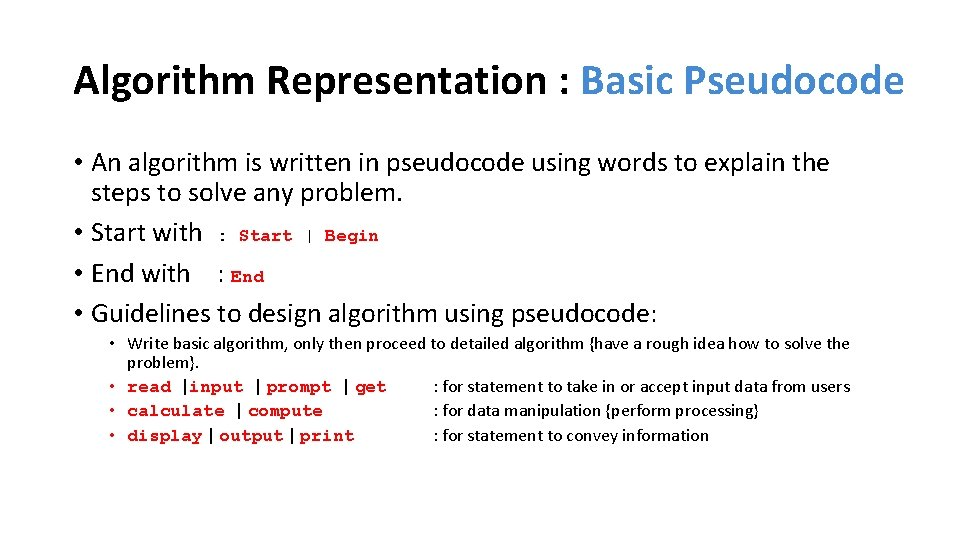Algorithm Representation : Basic Pseudocode • An algorithm is written in pseudocode using words to explain the steps to solve any problem. • Start with : Start | Begin • End with : End • Guidelines to design algorithm using pseudocode: • Write basic algorithm, only then proceed to detailed algorithm {have a rough idea how to solve the problem}. • read |input | prompt | get : for statement to take in or accept input data from users • calculate | compute : for data manipulation {perform processing} • display | output | print : for statement to convey informationAlgorithm Representation: Flowchart Name Symbol Description Lozenge Indicates the starting or ending of flowchart (start / stop : terminal ) Connector Used to connect break in the flowchart Parallelelogram Use for input and output operation (data) Rectangle Indicates any interval operation (process) Diamond Use for asking questions that can have either TRUE of FALSE / YES or NO (decision / selection) Module Sub solution to solve a part of entire problem Control flow Shows flow direction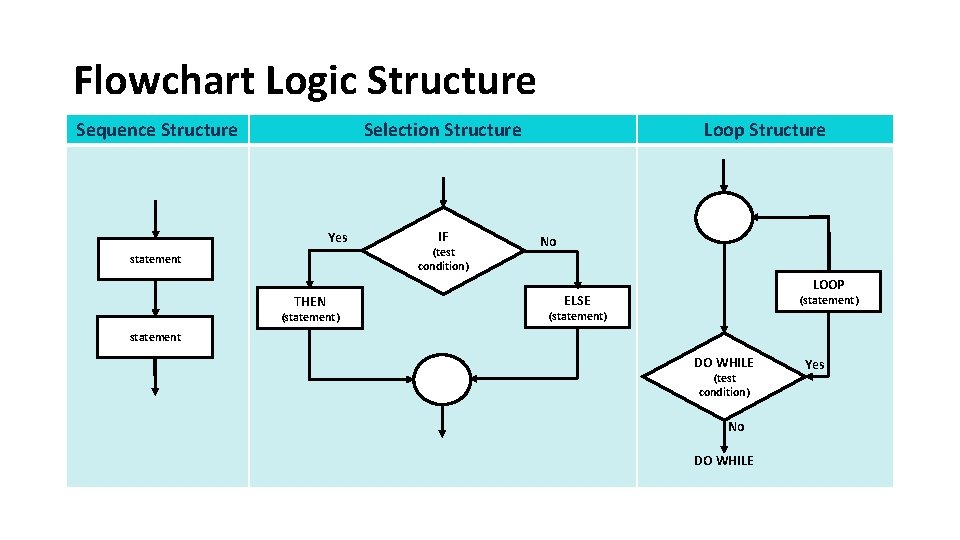Flowchart Logic Structure Sequence Structure Selection Structure Yes statement THEN (statement) IF (test condition) Loop Structure No LOOP ELSE (statement) statement DO WHILE (test condition) No DO WHILE Yes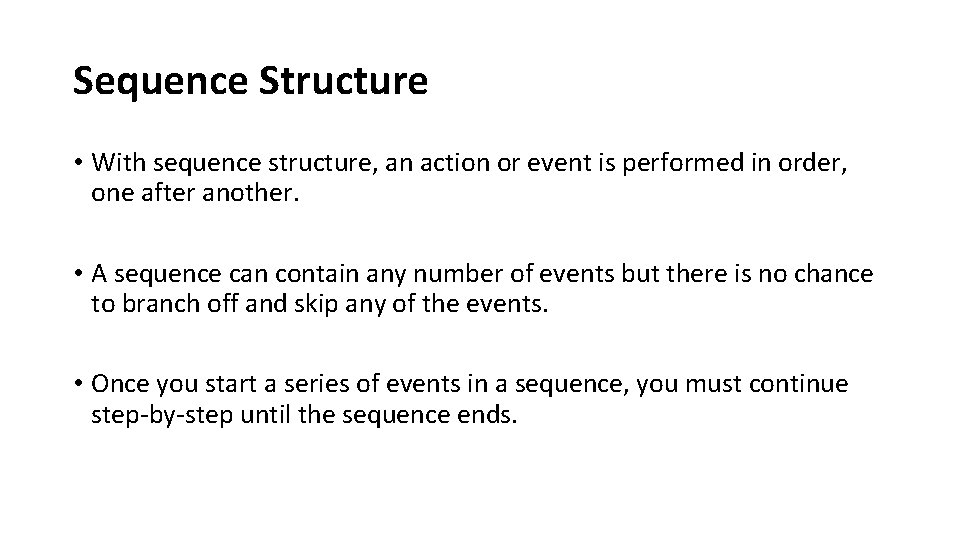Sequence Structure • With sequence structure, an action or event is performed in order, one after another. • A sequence can contain any number of events but there is no chance to branch off and skip any of the events. • Once you start a series of events in a sequence, you must continue step‐by‐step until the sequence ends.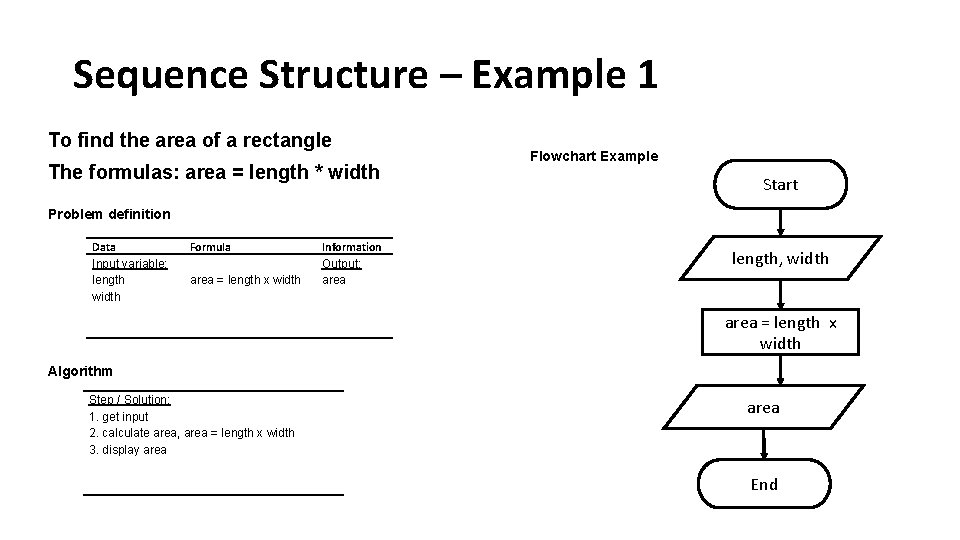Sequence Structure – Example 1 To find the area of a rectangle The formulas: area = length * width Flowchart Example Start Problem definition Data Input variable: length width Formula area = length x width Information Output: area length, width area = length x width Algorithm Step / Solution: 1. get input 2. calculate area, area = length x width 3. display area End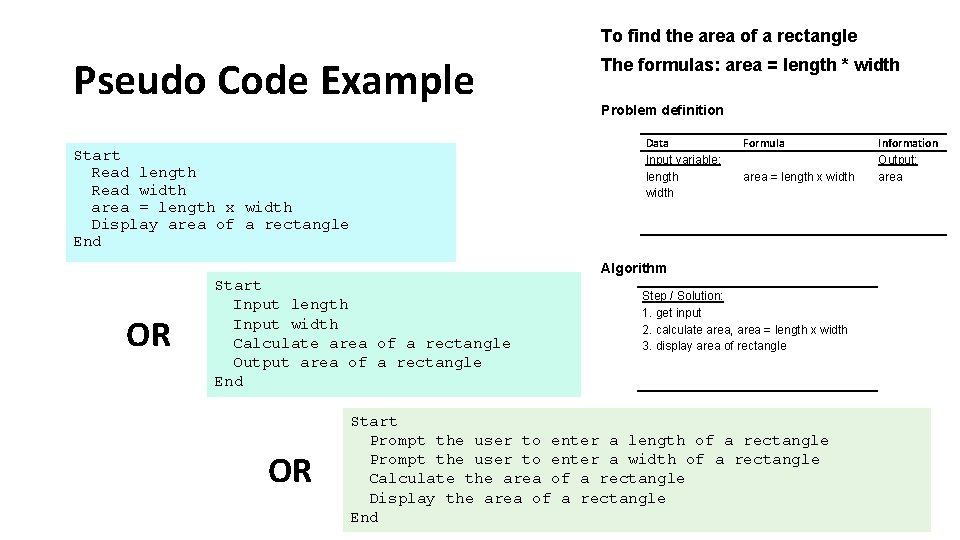To find the area of a rectangle Pseudo Code Example The formulas: area = length * width Problem definition Data Input variable: length width Start Read length Read width area = length x width Display area of a rectangle End Formula area = length x width Algorithm OR Start Input length Input width Calculate area of a rectangle Output area of a rectangle End OR Step / Solution: 1. get input 2. calculate area, area = length x width 3. display area of rectangle Start Prompt the user to enter a length of a rectangle Prompt the user to enter a width of a rectangle Calculate the area of a rectangle Display the area of a rectangle End Information Output: area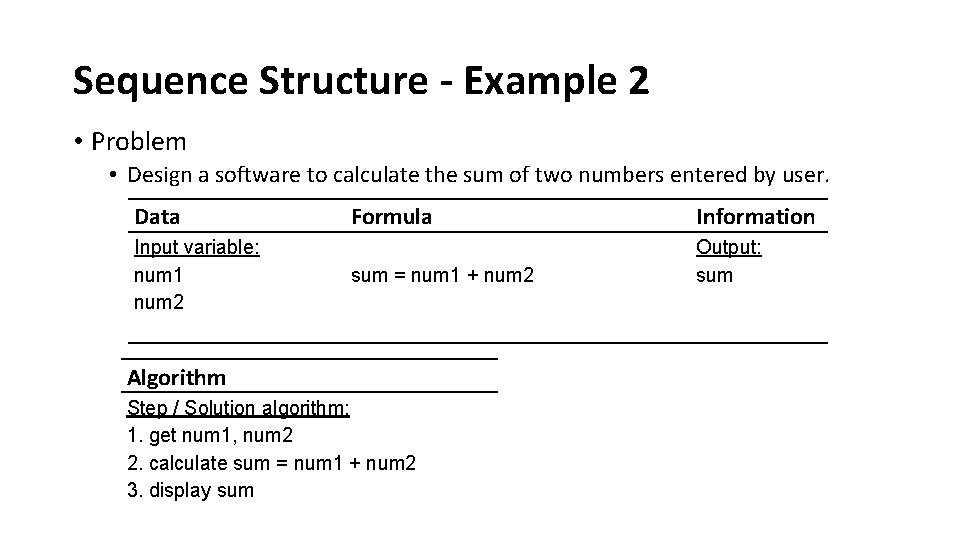Sequence Structure - Example 2 • Problem • Design a software to calculate the sum of two numbers entered by user. Data Input variable: num 1 num 2 Formula Information sum = num 1 + num 2 Output: sum Algorithm Step / Solution algorithm: 1. get num 1, num 2 2. calculate sum = num 1 + num 2 3. display sumSequence Example Pseudo Code Simple Design Modular Design Start End Read num 1 Read num 2 Calculate sum = num 1 + num 2 Print sum End get_input() calculate_sum() display_output() Module get_input() Read num 1 Read num 2 Return Module calculate_sum() sum = num 1 + num 2 Return Module display_output() Print sum Return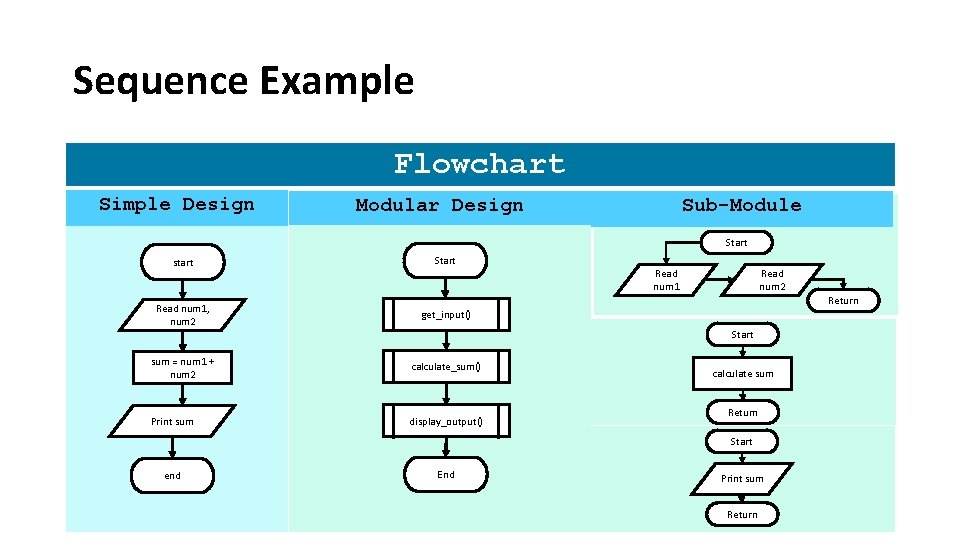Sequence Example Flowchart Simple Design Modular Sub-Module Design Start start Start Read num 1, num 2 get_input() sum = num 1 + num 2 calculate_sum() Print sum display_output() Read num 1 Read num 2 Return Start calculate sum Return Start end End Print sum ReturnWhy Algorithm is Important? • Describe the steps needed to perform a computation • Important for writing efficient code • code that execute faster & which uses less memory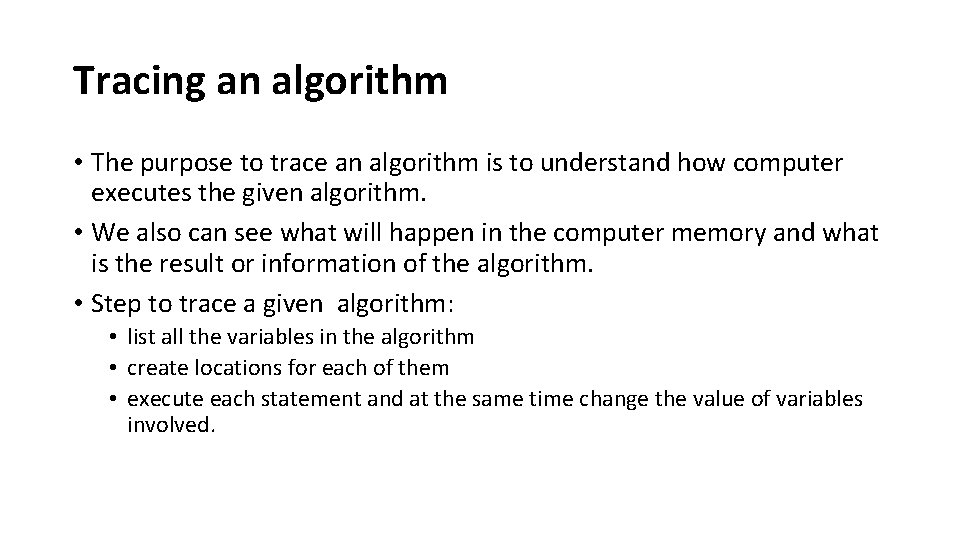Tracing an algorithm • The purpose to trace an algorithm is to understand how computer executes the given algorithm. • We also can see what will happen in the computer memory and what is the result or information of the algorithm. • Step to trace a given algorithm: • list all the variables in the algorithm • create locations for each of them • execute each statement and at the same time change the value of variables involved.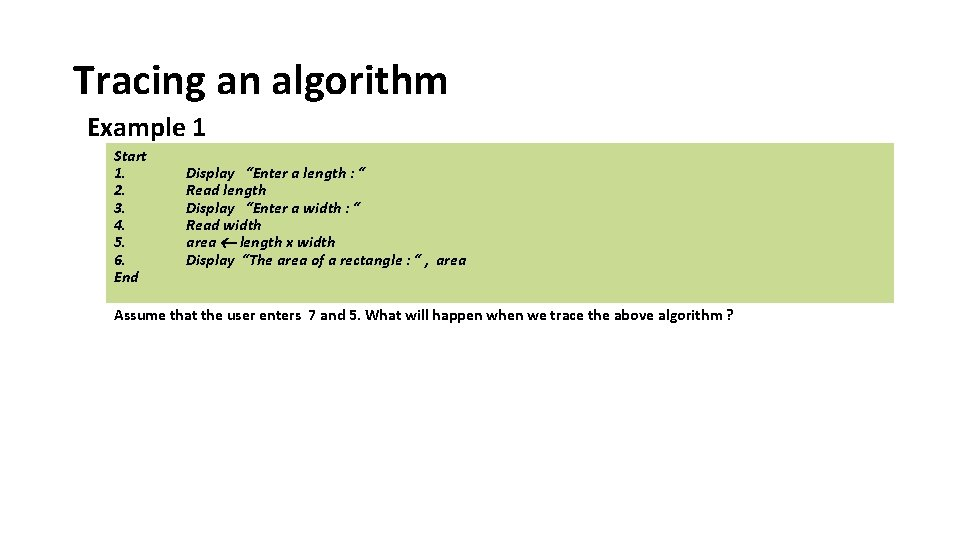Tracing an algorithm Example 1 Start 1. 2. 3. 4. 5. 6. End Display “Enter a length : “ Read length Display “Enter a width : “ Read width area length x width Display “The area of a rectangle : “ , area Assume that the user enters 7 and 5. What will happen when we trace the above algorithm ?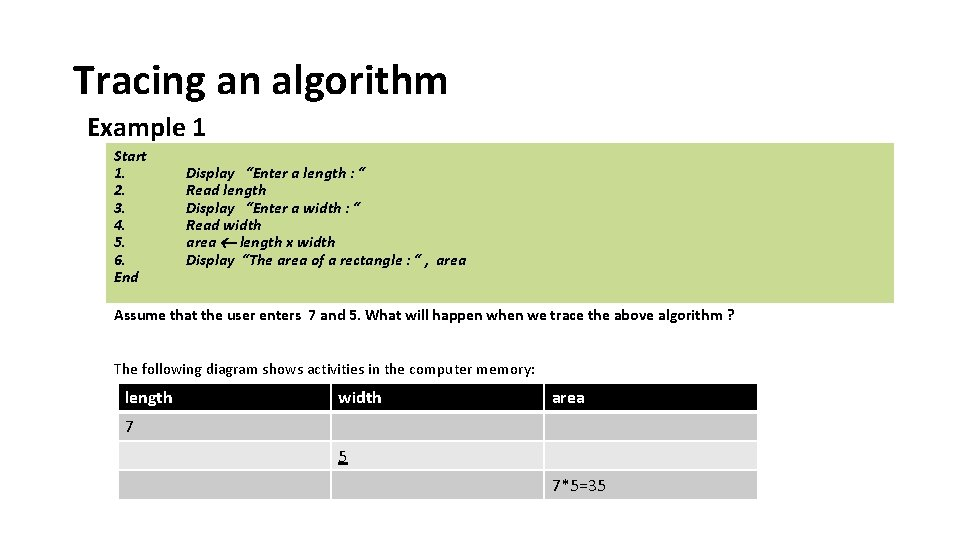Tracing an algorithm Example 1 Start 1. 2. 3. 4. 5. 6. End Display “Enter a length : “ Read length Display “Enter a width : “ Read width area length x width Display “The area of a rectangle : “ , area Assume that the user enters 7 and 5. What will happen when we trace the above algorithm ? The following diagram shows activities in the computer memory: length width area 7 5 7*5=35Tracing an algorithm Example 2 Start 1. Display “Enter marks for 3 tests : ” 2. Read test 1, test 2, test 3 3. Display ” Enter marks for 4 quizzes : ” 4. Read qz 1, qz 2, qz 3, qz 4 5. Display ” Enter final exam mark : ” 6. Read final-exam 7. test-mark ( test 1 + test 2 + test 3)/3 8. quiz-mark ( qz 1 + qz 2 + qz 3 + qz 4 )/4 9. carry-mark 20% x test-mark + 20% x quiz-mark 10. final-mark carry-mark + 60% x final-exam 11. Display message “Final mark : “, final-mark End Assume that the user enters 60 90 90 60 80 70 80 90. What will happen when we trace the above algorithm ?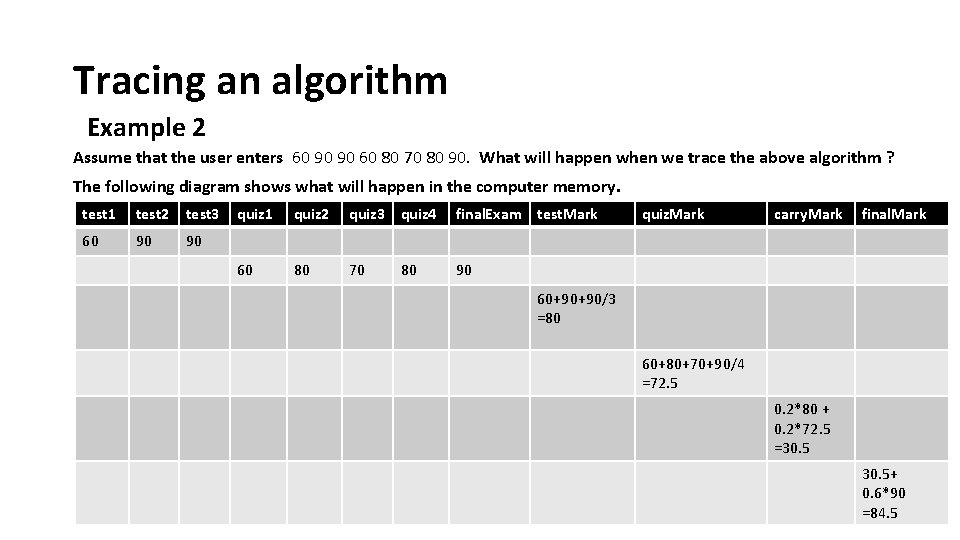Tracing an algorithm Example 2 Assume that the user enters 60 90 90 60 80 70 80 90. What will happen when we trace the above algorithm ? The following diagram shows what will happen in the computer memory. test 1 test 2 test 3 60 90 90 quiz 1 quiz 2 quiz 3 quiz 4 final. Exam test. Mark 60 80 70 90 80 quiz. Mark carry. Mark final. Mark 60+90+90/3 =80 60+80+70+90/4 =72. 5 0. 2*80 + 0. 2*72. 5 =30. 5+ 0. 6*90 =84. 5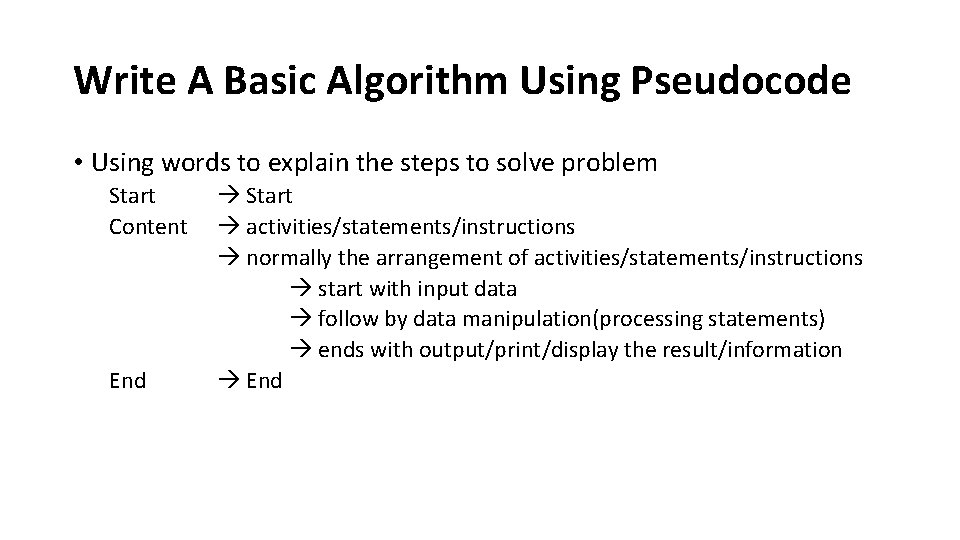Write A Basic Algorithm Using Pseudocode • Using words to explain the steps to solve problem Start Content End Start activities/statements/instructions normally the arrangement of activities/statements/instructions start with input data follow by data manipulation(processing statements) ends with output/print/display the result/information End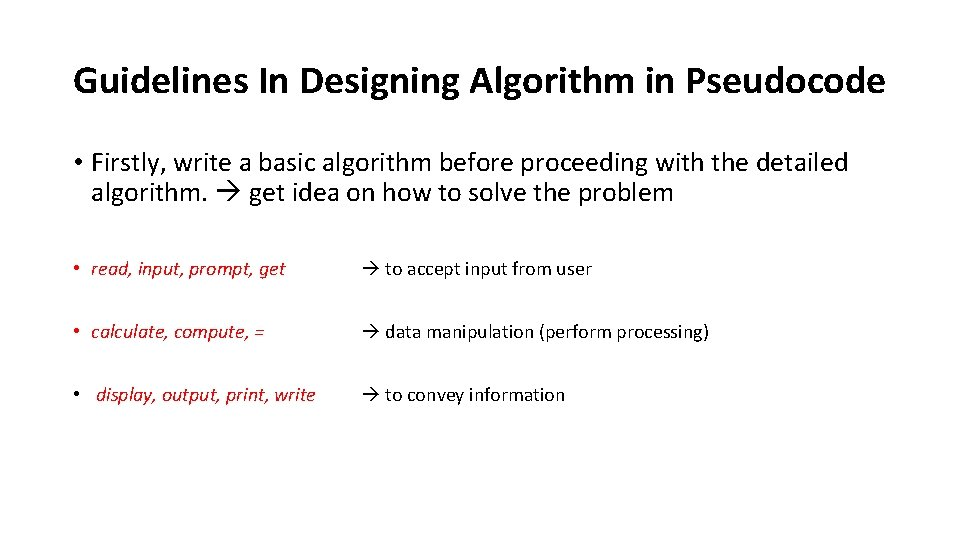Guidelines In Designing Algorithm in Pseudocode • Firstly, write a basic algorithm before proceeding with the detailed algorithm. get idea on how to solve the problem • read, input, prompt, get to accept input from user • calculate, compute, = data manipulation (perform processing) • display, output, print, write to convey informationEXERCISES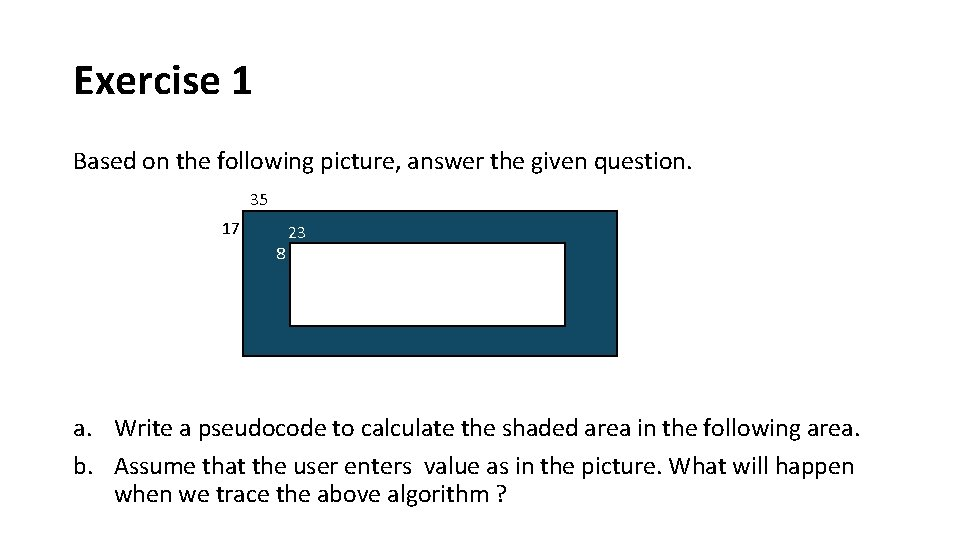Exercise 1 Based on the following picture, answer the given question. 35 17 8 23 a. Write a pseudocode to calculate the shaded area in the following area. b. Assume that the user enters value as in the picture. What will happen when we trace the above algorithm ?